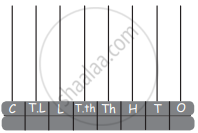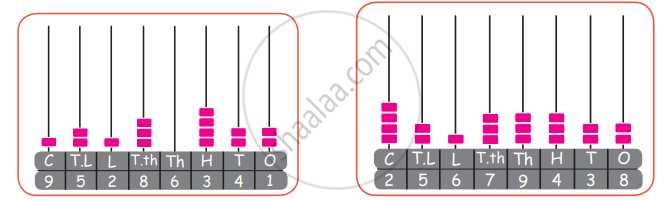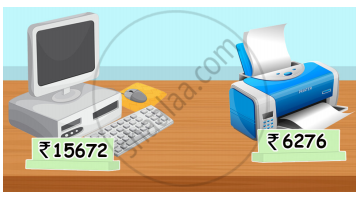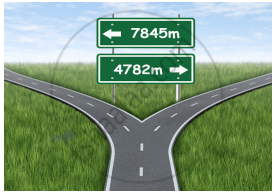Tamil Nadu Board of Secondary EducationSSLC (English Medium) Class 5th

# Tamil Nadu Board Samacheer Kalvi solutions for Class 5th Mathematics Term 1 Answers Guide chapter 2 - Numbers [Latest edition]

#### Chapters## Chapter 2: Numbers

Exercise 2.1Exercise 2.2Exercise 2.3Exercise 2.4Exercise 2.5Exercise 2.6Exercise 2.7Exercise 2.8Exercise 2.9
Exercise 2.1 [Page 26]

### Tamil Nadu Board Samacheer Kalvi solutions for Class 5th Mathematics Term 1 Answers Guide Chapter 2 Numbers Exercise 2.1 [Page 26]

#### Fill in the blanks

Exercise 2.1 | Q 1. a) | Page 26

10101; 10102; 10103; __________; ___________; ___________; ____________

Exercise 2.1 | Q 1. b) | Page 26

10220; 10230; __________; ___________; ___________; 10270

Exercise 2.1 | Q 1. c) | Page 26

10920; ________; ________; ________; 10960; ________

Exercise 2.1 | Q 1. d) | Page 26

11101; 11102; 11103; ________; ________; ________; ________

Exercise 2.2 [Page 31]

### Tamil Nadu Board Samacheer Kalvi solutions for Class 5th Mathematics Term 1 Answers Guide Chapter 2 Numbers Exercise 2.2 [Page 31]

Exercise 2.2 | Q 1. a. | Page 31

Consider the number 15,478

The place value of 7 is ______________

Exercise 2.2 | Q 1. b. | Page 31

Consider the number 15,478

The place value of 4 is ____________

Exercise 2.2 | Q 1. c. | Page 31

Consider the number 15,478

The place value of 1 is _____________

Exercise 2.2 | Q 2 | Page 31

Fill the table with the place value for the following numbers.

 Place value core lakhs thousands ones Numbers 1,00,00,000 10,00,000 1,00,000 10000 1000 100 10 1 23,45,172 2 3 4 5 1 7 2 84,701 2,01,784 9,04,704 9 0 4 7 0 4 2,07,91,132 10,07,000
Exercise 2.2 | Q 3 | Page 31

Find the difference between greatest 7-digit number and smallest 6-digit number

Exercise 2.3 [Page 32]

### Tamil Nadu Board Samacheer Kalvi solutions for Class 5th Mathematics Term 1 Answers Guide Chapter 2 Numbers Exercise 2.3 [Page 32]

Exercise 2.3 | Q 1. a. | Page 32

Read the following numbers by placing the commas at appropriate periods and write their number name.

15731997

Exercise 2.3 | Q 1. b. | Page 32

Read the following numbers by placing the commas at appropriate periods and write their number name.

341964

Exercise 2.3 | Q 1. c. | Page 32

Read the following numbers by placing the commas at appropriate periods and write their number name.

21921972

Exercise 2.3 | Q 1. d. | Page 32

Read the following numbers by placing the commas at appropriate periods and write their number name.

347810

Exercise 2.3 | Q 2. a. | Page 32

Write the place value of 5 in the following numbers

287500

Exercise 2.3 | Q 2. b. | Page 32

Write the place value of 5 in the following numbers

586012

Exercise 2.3 | Q 2. c. | Page 32

Write the place value of 5 in the following numbers

5869732

Exercise 2.3 | Q 2. d. | Page 32

Write the place value of 5 in the following numbers

5467859

Exercise 2.3 | Q 3. a. | Page 32

Write the following numbers in standard notation

30000 + 3000 + 300 + 30 + 3

Exercise 2.3 | Q 3. b. | Page 32

Write the following numbers in standard notation.

200000 + 7000 + 7

Exercise 2.3 | Q 3. c. | Page 32

Write the following numbers in standard notation.

8000000 + 70000 + 3000 + 30 + 5

Exercise 2.3 | Q 3. d. | Page 32

Write the following numbers in standard notation.

4000000 + 400 + 4

Exercise 2.3 | Q 4. a. | Page 32

Write the following numbers in expanded from.

63,570

Exercise 2.3 | Q 4. b. | Page 32

Write the following numbers in expanded from.

36,01,478

Exercise 2.3 | Q 4. c. | Page 32

Write the following numbers in expanded from.

1,45,70,004

Exercise 2.3 | Q 4. d. | Page 32

Write the following numbers in expanded from.

28,48,387

Exercise 2.4 [Pages 36 - 37]

### Tamil Nadu Board Samacheer Kalvi solutions for Class 5th Mathematics Term 1 Answers Guide Chapter 2 Numbers Exercise 2.4 [Pages 36 - 37]

Exercise 2.4 | Q 1. a. | Page 36

Write the number name

11000 _____________________

Exercise 2.4 | Q 1. b. | Page 36

Write the number name

34000 __________________________________

Exercise 2.4 | Q 1. c. | Page 36

Write the number name

100000 __________________________________

Exercise 2.4 | Q 1. d. | Page 36

Write the number name

98,364 ________________________

Exercise 2.4 | Q 1. e. | Page 36

Write the number name

37,689 __________________________________

Exercise 2.4 | Q 1. f. | Page 36

Write the number name

46,763 _____________________________

Exercise 2.4 | Q 1. g. | Page 36

Write the number name

4,00,000 __________________________________

Exercise 2.4 | Q 1. h. | Page 36

Write the number name

12,00,000 __________________________________

Exercise 2.4 | Q 2. a. | Page 36

Represent given values in Abacus.

3 Ten crores, 7 crores, 6 ten lakhs, 7 lakhs, 4 tens and 7 ones.Exercise 2.4 | Q 2. b. | Page 36

Represent given values in Abacus.

Find the place value of 7 and 4 of this numbers 34578910Exercise 2.4 | Q 2. c. a. | Page 36

Represent given values in Abacus.

Write in numeral: One crore forty thousand and four.

Exercise 2.4 | Q 2. c. b. | Page 36

Represent given values in Abacus.

Write in numeral: Sixty four lakhs and three

Exercise 2.4 | Q 2. d. | Page 37

Represent given values in Abacus.

Write the number name of the following numbers represented in the AbacusExercise 2.4 | Q 2. e. | Page 37

Represent given values in Abacus.

How many lakhs and hundreds are there in the numbers represented by the given AbacusExercise 2.4 | Q 2. f. | Page 37

Represent given values in Abacus.

Find the sum of greatest 4-digit number and smallest 5-digit numbers

Exercise 2.4 | Q 2. g. a | Page 37

Represent given values in Abacus.

Write in ascending order and descending order.

33,058, 40,978, 97,879, 81,421, 90,470, 47,224

Exercise 2.4 | Q 2. g. b. | Page 37

Represent given values in Abacus.

Write the following ascending and descending order.

99,999, 11,111, 22,222, 33,333, 44,444, 66,666

Exercise 2.4 | Q 2. h. | Page 37

Represent given values in Abacus.

Write in standard form: 7 lakhs + 5 thousands + 4 tens + 3 ones

Exercise 2.4 | Q 2. i. | Page 37

Represent given values in Abacus.

Add 5 thousands and 3 hundreds to this number 1,34,510

Exercise 2.4 | Q 2. j. | Page 37

Represent given values in Abacus.

Subtract smallest 6-digit numbers from greatest 7-digit numbers.

Exercise 2.5 [Page 39]

### Tamil Nadu Board Samacheer Kalvi solutions for Class 5th Mathematics Term 1 Answers Guide Chapter 2 Numbers Exercise 2.5 [Page 39]

Exercise 2.5 | Q 1. a. | Page 39

Find the sum.

6 8 7 5
6 3 7
+ 5 4 3 0 0
5 4
___________

Exercise 2.5 | Q 1. b. | Page 39

Find the sum.

3 2 5 6 7
7 8
+ 4 3 2 4
5 0 0 0
__________

Exercise 2.5 | Q 2. a. | Page 39

19732 + 24105 + 525 + 48

Exercise 2.5 | Q 2. b. | Page 39

241605 + 34788 + 5003 + 2052

Exercise 2.5 | Q 2. c. | Page 39

1000 + 250787 + 3574 + 43

Exercise 2.5 | Q 2. d. | Page 39

7 + 65 + 324 + 52342

Exercise 2.5 | Q 3 | Page 39

Population of five villages in a town panchayat are 980; 3254; 4125; 687; 6786. What is the total population.

Exercise 2.5 | Q 4 | Page 39

Ramu bought some household things. The price list is given below. Find the total cost?

Fan            = ₹   3,250
Fridge       = ₹  26,437
Television  = ₹ 18,520
Iron box    = ₹      940
Cat            = ₹ 15,520

Exercise 2.5 | Q 5 | Page 39

A day’s sales of different vegetables in a vegetable shop is as follows: Brinjal ₹ 4500 Tomato ₹ 7800 Onion ₹ 26500 Potato ₹ 7825 and Beetroot ₹ 825 Find the total amount of sales

Exercise 2.6 [Page 41]

### Tamil Nadu Board Samacheer Kalvi solutions for Class 5th Mathematics Term 1 Answers Guide Chapter 2 Numbers Exercise 2.6 [Page 41]

Exercise 2.6 | Q a. 1) | Page 41

Subtract

78,347
(−) 59,475

Exercise 2.6 | Q a. 2) | Page 41

Subtract

67,056
(−)  3,748

Exercise 2.6 | Q a. 3) | Page 41

Subtract

1,58,376
(−)    47,978

Exercise 2.6 | Q a. 4) | Page 41

Subtract

89,700
(−)   4,538

Exercise 2.6 | Q b. | Page 41

Rahul has 3289 stamps. Ravi has 4021 stamps. How many more stamps does Ravi have than Rahul?

Exercise 2.6 | Q c. | Page 41

Create the story problem by using the pictures given below:Exercise 2.6 | Q c. | Page 41

Create the story problem by using the pictures given below:Exercise 2.7 [Page 44]

### Tamil Nadu Board Samacheer Kalvi solutions for Class 5th Mathematics Term 1 Answers Guide Chapter 2 Numbers Exercise 2.7 [Page 44]

Exercise 2.7 | Q 1. a. | Page 44

Multiply:

473 × 48

Exercise 2.7 | Q 1. b. | Page 44

Multiply:

4052 × 19

Exercise 2.7 | Q 1. c. | Page 44

Multiply:

876 × 25

Exercise 2.7 | Q 1. d. | Page 44

Multiply:

854 × 21

Exercise 2.7 | Q 1. e. | Page 44

Multiply:

417 × 39

Exercise 2.7 | Q 1. f. | Page 44

Multiply:

870 × 28

Exercise 2.7 | Q 2. a. | Page 44

There are 55 mangoes in a basket. Cost of one mango is ₹ 15. What is the total cost of 55 mangoes?

Exercise 2.7 | Q 2. b. | Page 44

There are 55 passengers in a bus. Each of them get tickets of ₹ 25. what is the amount collected by the conductor?

Exercise 2.7 | Q 2. c. | Page 44

A classroom has 23 benches, cost of one bench is ₹ 725. What is the total cost of all the 23 benches?

Exercise 2.7 | Q 2. d. | Page 44

There are 675 people living in a village. One person uses 25 L of water daily. How much of water is needed for the village for one day?

Exercise 2.7 | Q 2. e. | Page 44

In a building, there are 26 rooms, cost of painting for one room is ₹ 950 What is the total cost of painting the building?

Exercise 2.8 [Page 46]

### Tamil Nadu Board Samacheer Kalvi solutions for Class 5th Mathematics Term 1 Answers Guide Chapter 2 Numbers Exercise 2.8 [Page 46]

Exercise 2.8 | Q 1. a. | Page 46

Find quotient and remainder:

5732 ÷ 9

Exercise 2.8 | Q 1. b. | Page 46

Find quotient and remainder:

47345 ÷ 5

Exercise 2.8 | Q 1. c. | Page 46

Find quotient and remainder:

3032 ÷ 7

Exercise 2.8 | Q 1. d. | Page 46

Find quotient and remainder:

43251 ÷ 10

Exercise 2.8 | Q 1. e. | Page 46

Find quotient and remainder:

2532 ÷ 4

Exercise 2.8 | Q 2. a. | Page 46

3057 families are living in a town. The town panchayat decided to split the town into 3 wards. How many families will be there in each panchayat?

Exercise 2.8 | Q 2. b. | Page 46

A water board distributes 28,049 litres daily to a town in 7 lorries. How much of water will each lorry carry?

Exercise 2.8 | Q 2. c. | Page 46

A company gives ₹ 93,300 as salary for 6 workers equally. How much salary will each worker get?

Exercise 2.9 [Page 50]

### Tamil Nadu Board Samacheer Kalvi solutions for Class 5th Mathematics Term 1 Answers Guide Chapter 2 Numbers Exercise 2.9 [Page 50]

Exercise 2.9 | Q 1. a. | Page 50

A cement factory produces 37500 bags of cements in a month (30 days). How many cement bags were produced in one day?

Exercise 2.9 | Q 1. b. | Page 50

8075 mangoes were harvested from a mango garden. 95 mangoes are packed in each package. How many packages will be there?

Exercise 2.9 | Q 1. c. | Page 50

25 families living in a street needed 1625 liters of water per day. How much of water is needed by one family?

Exercise 2.9 | Q 1. d. | Page 50

6750 bananas have to be loaded in a tempo van. If 15 bananas are arranged in one basket, then how many baskets will be needed to arrange all the bananas?

Exercise 2.9 | Q 2. a. | Page 50

Divide the following

4525 ÷ 15

Exercise 2.9 | Q 2. b. | Page 50

Divide the following

3448 ÷ 24

Exercise 2.9 | Q 2. c. | Page 50

Divide the following

7342 ÷ 18

Exercise 2.9 | Q 2. d. | Page 50

Divide the following

3626 ÷ 37

Exercise 2.9 | Q 2. e. | Page 50

Divide the following

4872 ÷ 56

## Chapter 2: Numbers

Exercise 2.1Exercise 2.2Exercise 2.3Exercise 2.4Exercise 2.5Exercise 2.6Exercise 2.7Exercise 2.8Exercise 2.9## Tamil Nadu Board Samacheer Kalvi solutions for Class 5th Mathematics Term 1 Answers Guide chapter 2 - Numbers

Tamil Nadu Board Samacheer Kalvi solutions for Class 5th Mathematics Term 1 Answers Guide chapter 2 (Numbers) include all questions with solution and detail explanation. This will clear students doubts about any question and improve application skills while preparing for board exams. The detailed, step-by-step solutions will help you understand the concepts better and clear your confusions, if any. Shaalaa.com has the Tamil Nadu Board of Secondary Education Class 5th Mathematics Term 1 Answers Guide solutions in a manner that help students grasp basic concepts better and faster.

Further, we at Shaalaa.com provide such solutions so that students can prepare for written exams. Tamil Nadu Board Samacheer Kalvi textbook solutions can be a core help for self-study and acts as a perfect self-help guidance for students.

Concepts covered in Class 5th Mathematics Term 1 Answers Guide chapter 2 Numbers are Introducing a 5 Digit Number - 10,000, Introducing the Six Digit Number - 1,00,000, Larger Number of Digits 7 and Above, Revisiting Place Value of Numbers, Expansion Form of Numbers, Using Commas in Indian and International Number System, Comparing Numbers, Compare Numbers in Ascending and Descending Order, Number of Operation.

Using Tamil Nadu Board Samacheer Kalvi Class 5th solutions Numbers exercise by students are an easy way to prepare for the exams, as they involve solutions arranged chapter-wise also page wise. The questions involved in Tamil Nadu Board Samacheer Kalvi Solutions are important questions that can be asked in the final exam. Maximum students of Tamil Nadu Board of Secondary Education Class 5th prefer Tamil Nadu Board Samacheer Kalvi Textbook Solutions to score more in exam.

Get the free view of chapter 2 Numbers Class 5th extra questions for Class 5th Mathematics Term 1 Answers Guide and can use Shaalaa.com to keep it handy for your exam preparation